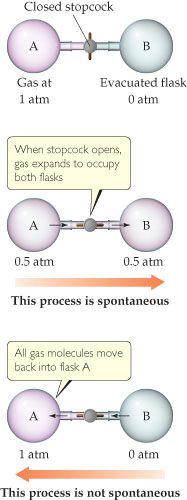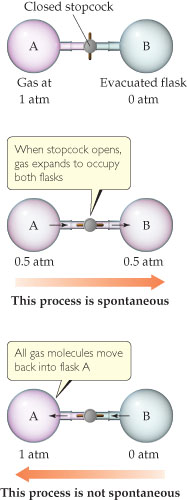Chemistry Practice Problems Chemistry Gas Laws Practice Problems Solution: Expansion of a gas into an evacuated space is a sp...

🤓 Based on our data, we think this question is relevant for Professor Rhandir's class at GCU.

# Solution: Expansion of a gas into an evacuated space is a spontaneous process. The reverse process-gas molecules initially distributed evenly in two flasks all moving into one flask-is not spontaneous.If flask B were smaller than flask A, would the final pressure after the stopcock is opened be greater than, equal to, or less than 0.5 atm?

###### ProblemExpansion of a gas into an evacuated space is a spontaneous process. The reverse process-gas molecules initially distributed evenly in two flasks all moving into one flask-is not spontaneous.

If flask B were smaller than flask A, would the final pressure after the stopcock is opened be greater than, equal to, or less than 0.5 atm?

###### Solution

We are given the following figure:We are asked if flask B were smaller than flask A, would the final pressure after the stopcock is opened be greater than, equal to, or less than 0.5 atm.

Recall that the ideal gas law is:

$\overline{){\mathbf{PV}}{\mathbf{=}}{\mathbf{nRT}}}$

The pressure and volume of a gas are related to the number of moles, gas constant and temperatureThe value nRT is constantView Complete Written Solution

Chemistry Gas Laws

Chemistry Gas Laws

#### Q. An ideal gas at a pressure of 1.60 atm is contained in a bulb of unknown volume. A stopcock is used to connect this bulb with a previously evacuated b...

Solved • Wed Oct 31 2018 14:12:50 GMT-0400 (EDT)

Chemistry Gas Laws

#### Q. A gas occupies a volume of 0.75 L at 20 oC at 720 torr. What volume would the gas occupy at 41 oC and 760 torr?

Solved • Wed Oct 31 2018 14:12:50 GMT-0400 (EDT)

Chemistry Gas Laws

#### Q. Amontons law expresses the relationship between pressure and temperature. Use Charless law and Boyles law to derive the proportionality relationship...

Solved • Wed Oct 31 2018 14:12:49 GMT-0400 (EDT)

Chemistry Gas Laws

#### Q. If a car tire is filled to a pressure of 32.0 lbs/in2 (psi) measured at 76 ˚F, what will be the tire pressure if the tires heat up to 121 ˚F during dr...

Solved • Wed Oct 31 2018 14:12:49 GMT-0400 (EDT)